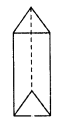# RD Sharma Class 8 Solutions Chapter 19 Visualising Shapes Ex 19.2

In this chapter, we provide RD Sharma Class 8 Solutions Chapter 19 Visualising Shapes Ex 19.2 for English medium students, Which will very helpful for every student in their exams. Students can download the latest RD Sharma Class 8 Solutions Chapter 19 Visualising Shapes Ex 19.2 Maths pdf, free RD Sharma Class 8 Solutions Chapter 19 Visualising Shapes Ex 19.2 book pdf download. Now you will get step by step solution to each question.

### RD Sharma Solutions for Class 8 Chapter 19 Visualising Shapes Ex 19.2Download PDF

Question 1.
Which among the following are nets for a cube ?Solution:
Nets for a cube are (ii), (iv) and (vi)

Question 2.
Name the polyhedron that can be made by folding each net:Solution:
(i) This net is for a square(ii) This net is for triangular prism.(iii) This net is for triangular prism.(iv) This net is for hexagonal prism.(v) This net is for hexagon pyramid.(vi) This net is for cuboid.Question 3.
Dice are cubes where the numbers on the opposite faces must total 7. Which of the following are dice ?Solution:
Figure (i) shows the net of cube or dice.

Question 4.
Draw nets for each of the following polyhedrons:Solution:
(i) Net for cube is given below :(ii) Net of a triangular prism is as under :(iii) Net of hexagonal prism is as under :(iv) The net for pentagonal pyramid is as under:Question 5.
Match the following figures:Solution:
(a) (iv)
(b) (i)
(c) (ii)
(d) (iii)

All Chapter RD Sharma Solutions For Class 8 Maths

—————————————————————————–

All Subject NCERT Exemplar Problems Solutions For Class 8

All Subject NCERT Solutions For Class 8

*************************************************

I think you got complete solutions for this chapter. If You have any queries regarding this chapter, please comment on the below section our subject teacher will answer you. We tried our best to give complete solutions so you got good marks in your exam.

If these solutions have helped you, you can also share rdsharmasolutions.in to your friends.